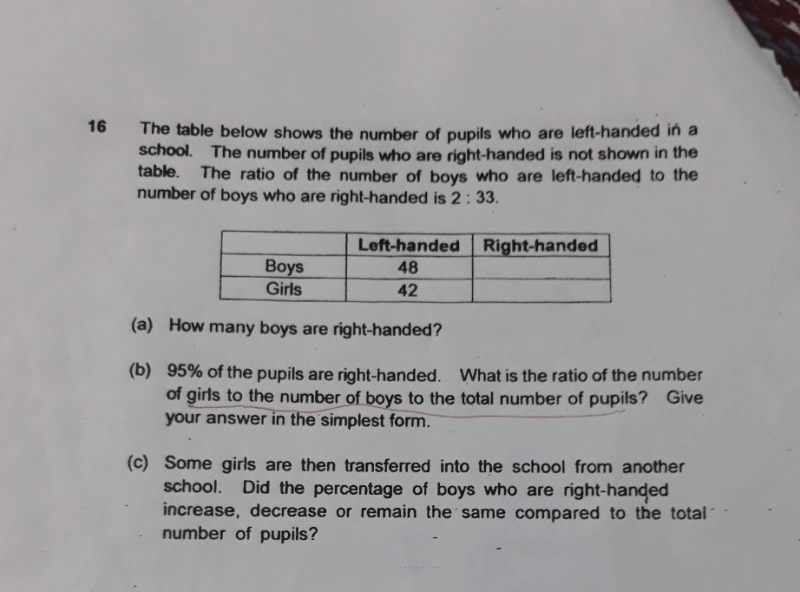# QuestionCan you please explain this question?

a) Boys RH : Boys LH = 2 : 33
48 ÷ 2 x 33 = 792 right-handed boys

b) Number of left-handed pupils → 48 + 42
= 90 left-handed pupils

Since 95% of students are right-handed,

5% = 90
1% = 18
100% = 1800 students in total

Total number of boys → 792 + 48
= 840 boys

Total number of girls → 1800 – 840
= 960 girls

Total no. of girls : Total no. of boys : Total number of pupils
960 : 840 : 1800
48 : 42 : 90
8 : 7 : 15

c) Since the total number of girls increased, this means the total number of pupils also increased.

So the total number of boys who are right-handed as a percentage of the total number of pupils will decrease.

0 Replies 0 Likes